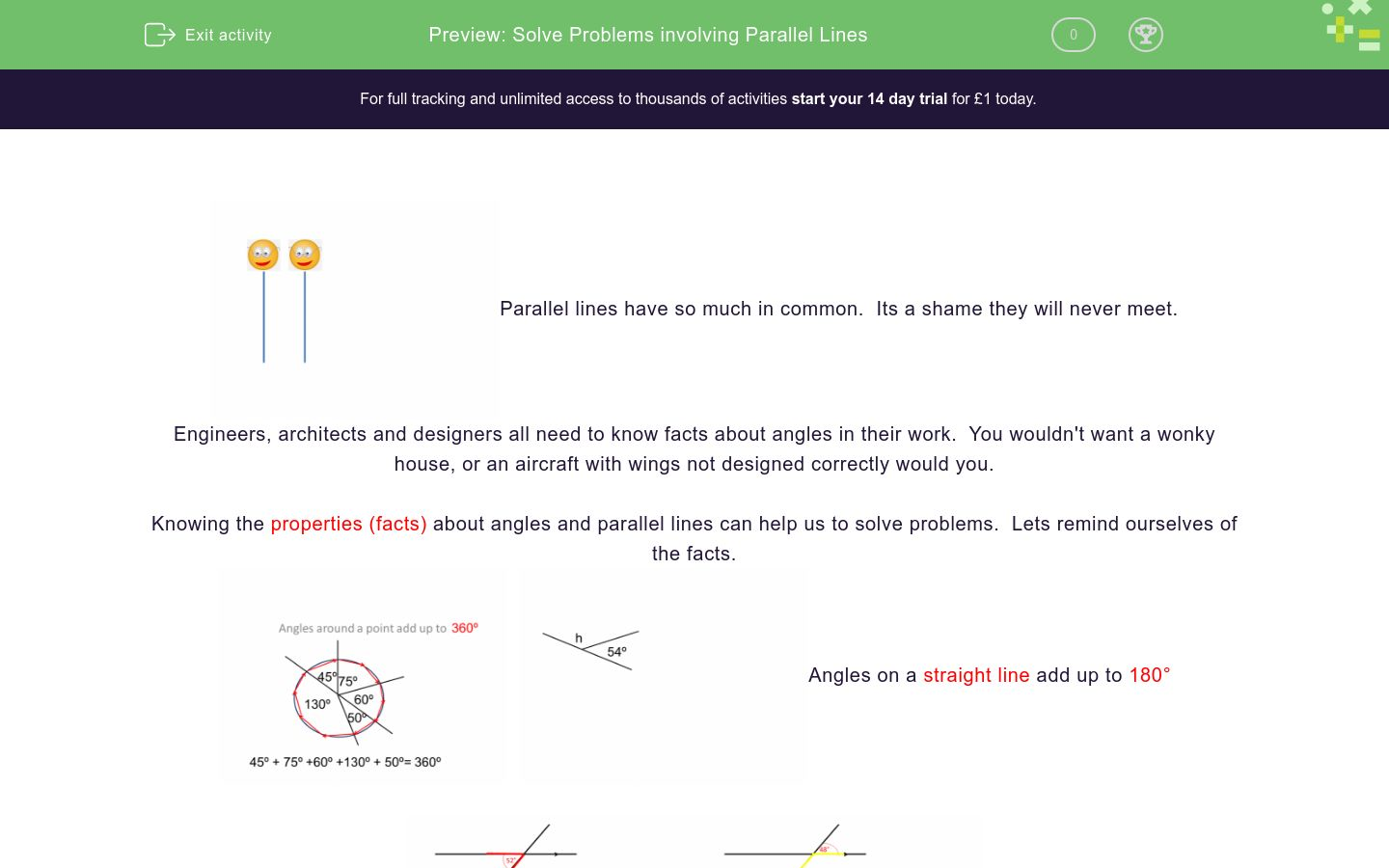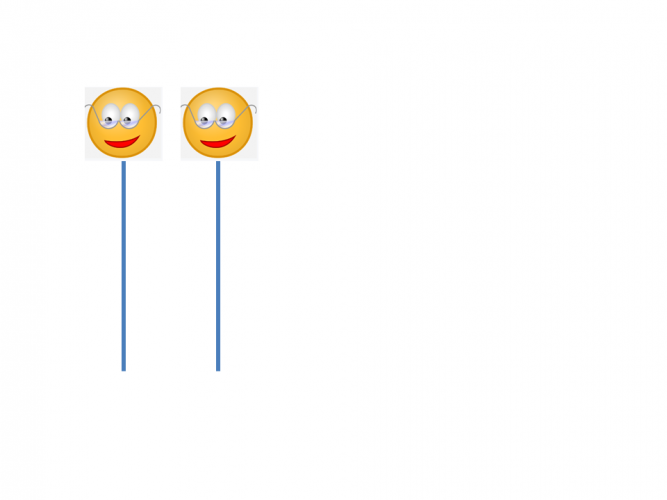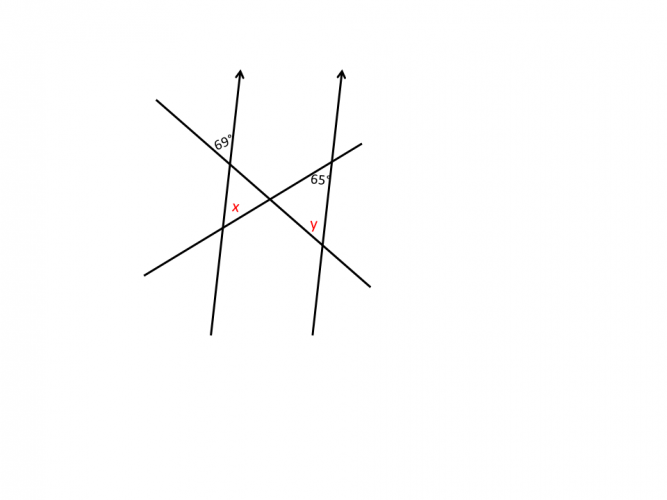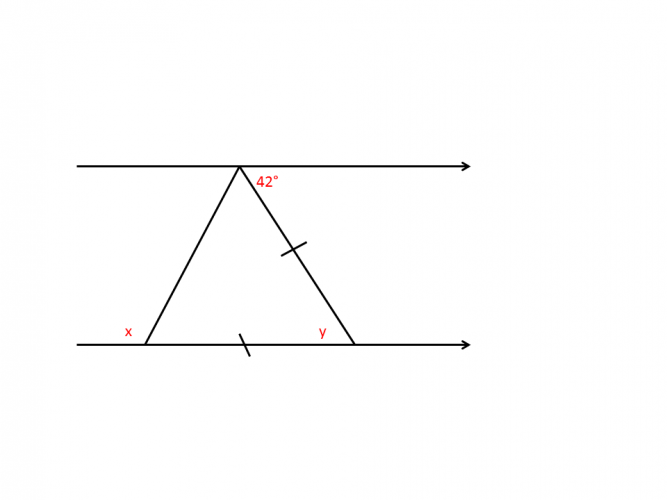# Solve Problems involving Parallel Lines

In this worksheet, students will combine their knowledge of angle properties of parallel lines to solve simple problems.Key stage:  KS 4

GCSE Subjects:   Maths

GCSE Boards:   Pearson Edexcel, AQA, Eduqas, OCR

Curriculum topic:   Geometry and Measures, Basic Geometry

Curriculum subtopic:   Properties and Constructions, Angles

Difficulty level:### QUESTION 1 of 10Parallel lines have so much in common.  Its a shame they will never meet.

Engineers, architects and designers all need to know facts about angles in their work.  You wouldn't want a wonky house, or an aircraft with wings not designed correctly would you.

Knowing the properties (facts) about angles and parallel lines can help us to solve problems.  Lets remind ourselves of the facts.Angles on a straight line add up to 180°Alternate angles are equal                              Corresponding angles are equalQuite often they are combined and so therefore you need an eagle eye to solve problems.

Lets give it a go.

Example 1

Find angle x.Here you need to draw in another parallel line to help.  There are no rules to say we can't do that.

You can now apply the alternate angle rules as shown.  28 + 38 = 66°

Example 2

Find the value of x and y.Y is a corresponding angle to x, therefore 114°

Angle x and y are on straight line, therefore x is 180 - 114 = 66°Find the value of x.

92°

48°

46°

88°134° 40° 180° The value of x is The value of y is The value of z is134° 40° 180° The value of x is The value of y is The value of z is134° 40° 180° The value of x is The value of y is The value of z isFind the value of y.Which two values represent x and y.

69°

111°

42°

120°108° 72° 36° 110° The value of x is The value of y isWhat is the value of x?The value of x is

177°

27°

130°

59°The value of x is 87° 93° 77°
• Question 1Find the value of x.

88°
EDDIE SAYS
Did you remember to draw in another parallel line. Once you do this you can see that alternate angles rule can be applied. 46 and 42 are now where x is so 46 + 42 = 88° How cool is that.
• Question 2134° 40° 180° The value of x is The value of y is The value of z is
EDDIE SAYS
x is alternate to 40° so, therefore, the same. The value of y is calculated by angles on a straight line. 180 - 46 = 134° z can be calculated in one of two ways. You may have spotted that it is corresponding to y, or that interior angles add up to 180. 180 - 46 = 134° And the beauty here is you can work things out in any order you want.
• Question 3EDDIE SAYS
Don't let two lines across the parallels put you off. x is alternate to 65° and y is corresponding to 69° No working out which is great as long as you know the rules.
• Question 4EDDIE SAYS
Two set of parallel lines here for the price of one. Apply interior angles add up to 180° to find x. 180 - 62 = 118° Apply this again to x and z. 180 - 118 = 62° y is alternate to 100° so therefore the same. Are you getting used to spotting different rules within the same diagram?
• Question 5Find the value of y.

64
EDDIE SAYS
Could you spot the alternate angle? Sometimes the sneaky teacher put in an angle that you don't need just to check that you know the rules. y = 64°
• Question 6Which two values represent x and y.

111°
42°
EDDIE SAYS
The sneaky part is that you need to find angles you are not asked for first. y is 42° as it is alternate to 42°. Look at the markings on the triangle. It shows that the triangle is isocelese. The two base angles are the same. You need a base angle to calculate x. 180 - 42 = 138° ÷2 = 69° x is on a straight line to 69° 180 - 69° = 111°
• Question 7108° 72° 36° 110° The value of x is The value of y is
EDDIE SAYS
Don't you just love it when they do this? Before you do anything you need to find the missing angles on the straight line with 2x and x. The missing angle is alternate to 72° Now to find x 180 - 72 = 108° Share the 108 between 3 =36° x is 36° y is alternate to x so also 36°
• Question 8What is the value of x?

50
EDDIE SAYS
Just use the parallel lines. The other base angle in the triangle is alternate to 52° Angles in a triangle add up to 180° 180 - 78 - 52 = 50°
• Question 9The value of x is

59°
EDDIE SAYS
Oh that wonderful curved ball we all love. No problems. We know that interior angles add up to 180°. 2x - 12 + x + 15 = 180° 3x + 3 = 180° 3x = 177° x = 59° Put this back into the original equation as it all adds up to 180°
• Question 10The value of x is 87° 93° 77°
EDDIE SAYS
You need to spot other angles that you are not asked to find here. The angle on the line next to x is corresponding to 87° Now we can work with angles on a straight line add up to 180° 180 - 87 = 93°
---- OR ----

Sign up for a £1 trial so you can track and measure your child's progress on this activity.

### What is EdPlace?

We're your National Curriculum aligned online education content provider helping each child succeed in English, maths and science from year 1 to GCSE. With an EdPlace account you’ll be able to track and measure progress, helping each child achieve their best. We build confidence and attainment by personalising each child’s learning at a level that suits them.

Get started